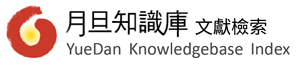1. 熱門：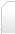首頁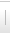臺灣期刊   法律   公行政治   醫學   財經   社會學   教育   其他大陸期刊   核心   非核心DOI文章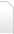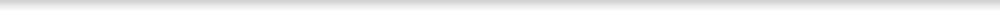篇名 並列篇名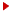查看詳細全文 數學素養導向的任務設計與教學實踐──以發展學童的數學論證為例 Task Design and Implementation for Mathematical Literacy: Developing Students’ Mathematical Argumentation 林碧珍、鄭章華、陳姿靜 本文旨在提供國小階段十二年國教素養導向任務設計與教學實踐之範例，作為教科書編撰者落實到數學教材設計之參考，並協助教師瞭解課堂中數學素養培養的作法。本文首先探討素養內涵中數學論證的意義與重要性；其次，以數學論證為教學目標及以四年級「認識各種三角形及其性質」為教學脈絡，設計數學臆測任務，來詮釋從官方到書面課程的轉化；並以教師在課堂中的教學實踐說明從書面到實踐課程的轉化。本文提出數學臆測是培養素養內涵中數學論證的途徑之一。數學臆測能幫助學童建立較嚴謹的數學知識，能引出一叢相關的數學性質或概念，能培養學童提出證據以取信他人，以及能舉出反駁的例子或證據推翻他人的觀點之民主素養。本文於文末提供建議給教科書編撰參考。 This paper provides an exemplar for textbook writers and teachers to design tasks for mathematical literacy and implementing them into classrooms. Mathematical literacy is the kernel focus of the current innovations of 12-year compulsory education. This paper begins by briefly introducing the significance and the meaning of mathematical argumentation. Then, a task design for conjecturing integrated into mathematics content with recognizing various triangles and its properties as an example to illustrate the meaning of transforming intended curriculum into written curriculum. It is suggested that mathematical conjecturing is an approach to evoke mathematical argumentation as one aspect of mathematical literacy. Mathematical conjecturing is an approach for illustrating mathematics as a language and mathematics as scientific pattern. Mathematical conjecturing has a power of construing a series of connected mathematics properties or mathematics concepts as an approach to enhancing mathematical literacy. Moreover, mathematics as a language and mathematics as a pattern of science are achieved via conjecturing. Students convince others via warrants and reject the arguments of others using rebuttals or they support others’ arguments via warrants or backing. This paper concludes with remarks for mathematical textbooks for textbook editors and teachers. 109-134 十二年國教、書面課程、數學領域、數學素養、數學論證、12-year compulsory education、written curriculum、mathematics learning area、mathematical literacy、mathematical argumentation 教科書研究 201604 (9:1期) 國家教育研究院 00417989  複製DOI該期刊-上一篇 他者的歷史殤慟──兩岸中學社會科教科書中猶太大屠殺議題之敘寫與啟思 該期刊-下一篇 英美日科技教科書分析及其對十二年國教之啟示

### 優惠活動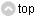月旦實務講座 ．講座試聽 ．進修課程 月旦影音論壇 月旦知識庫 ．數位授權 ．個人購點 ．單位採購 月旦醫事法網 期刊數位服務．DOI註冊 ．電子期刊 ．投審系統 ．學術不端檢測 社群平台 讀者服務 關於元照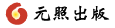讀者服務專線：+886-2-23756688　傳真：+886-2-23318496 地址：臺北市館前路28 號 7 樓　客服信箱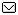Copyright © 元照出版 All rights reserved. 版權所有，禁止轉貼節錄